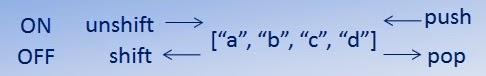## Monday, 28 July 2014

### Ruby - Array: Adding and removing data with Pop, Push, Shift, Unshift

In an earlier post we looked at basic Arrays and some tricks around delimited input (%W and %W etc.) What we saw were a few ways arrays could be built out and data added to them - when being created.

With that understanding however, we need to see how we'd add and remove data once the array is already set-up. Fortunately, Ruby provides a way to do that, albeit in a slightly cryptic way.

Let's say out array looks like this:

testArray = ["a""b""c""d"]

We'll want to do one of the following:
• REMOVE data from the START (Shift)
• ADD data to the START (Unshift)
• REMOVE data from the END (Pop)
• ADD data to the END (Push)
Graphically we can represent this as per the below:To experiment with this, let's set up and array then ask a user what they want to do with it. The array needs to be accessible in the If statement we're going to use in a second, so for each make it's scope up from local to instance by adding @

@testArray = ["one""two""three""four"]

puts "\nWhat would you like to do? (shift, unshift or push, pop)"
action = gets.chomp.downcase

Here the user can select one of the option of shift, unshift for working with the start of the array or push, pop for the end of the array.

Next let's build out an If statement framework;

if action == "pop"

elsif action == "push"

elsif action == "shift"

elsif action == "unshift"

end

Under each we then need to call the correct method for each action selected.

if action == "pop"
@testArray.pop

Can you complete the rest?

To help the user see what's going on, let's give a message about what's going to happen, then print out the contents of the array so we can see the result.

if action == "pop"
puts "\nPoping a value OFF the END of the array (#{@testArray[-1]}).\n"
@testArray.pop
puts "The current array looks like this:\n"
puts @testArray

Here we use some interpolation to return the end value before we remove it.

As above, have a go at writing the rest of the script for each of the methods.

Bonus

Using these methods, we don't have to modify one element at a time. We can specify the amount of elements to be modified by adding a value to the end of the method, for example;

@testArray.pop(2)

Mark.

---------------------------

@testArray = ["one", "two", "three", "four"]

puts @testArray

puts "\nWhat would you like to do? (pop, push or shift, unshift)"
action = gets.chomp.downcase

if action == "pop"
puts "\nPoping a value OFF the END of the array (#{@testArray[-1]}).\n"
@testArray.pop
puts "The current array looks like this:\n"
puts @testArray

elsif action == "push"
puts "\nPushing a value ONTO the END of the array.\n"
@testArray.push "five"
puts "The current array looks like this:\n"
puts @testArray

elsif action == "shift"
puts "\nShifting a value OFF the START of the array. (#{@testArray})\n"
@testArray.shift
puts "The current array looks like this:\n"
puts @testArray

elsif action == "unshift"
puts "Unshifting a value ONTO the START of the array.\n"
@testArray.unshift "zero"
puts "The current array looks like this:\n"
puts @testArray

else puts "That wasn't an option"

end